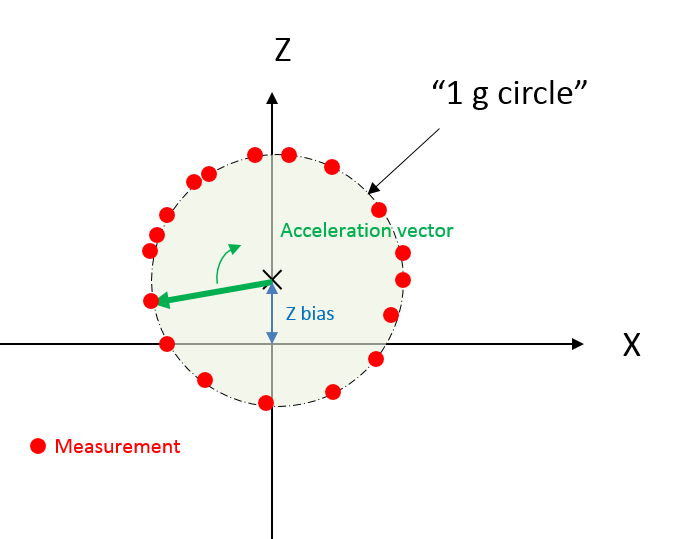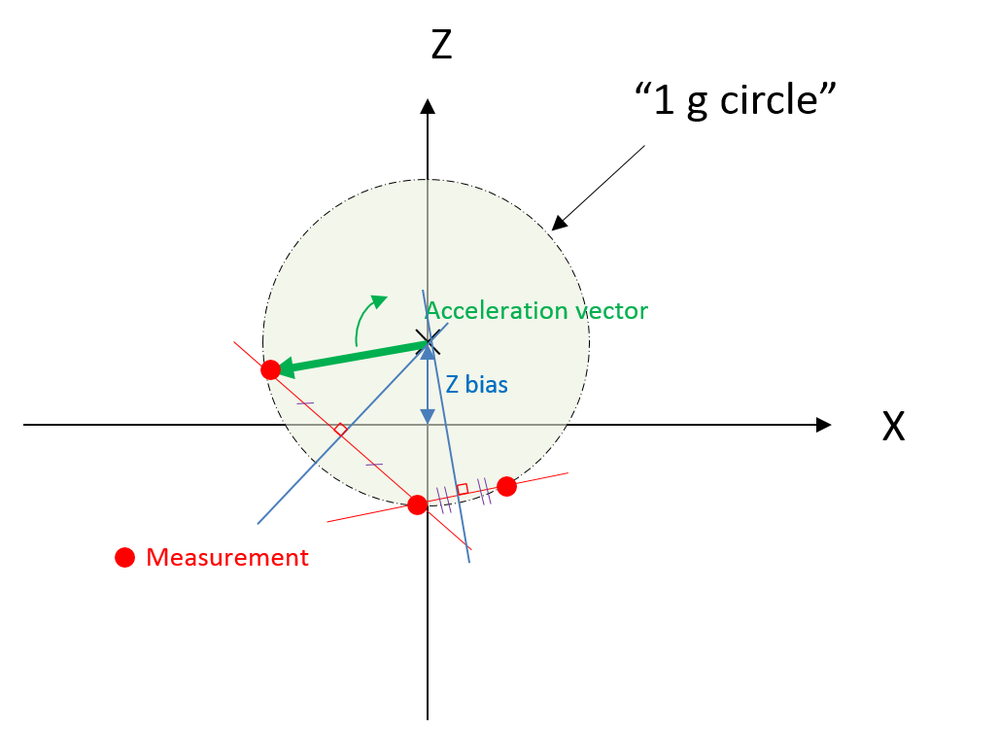# How to detect the Direction and Angle using XZ-accelerometer Sensor

cancel
Showing results for
Did you mean:

## How to detect the Direction and Angle using XZ-accelerometer Sensor

544 ViewsContributor III

Dear All,

I am using FXTH8719xxxx Family XZ axis Accelerometer Sensor. I need to find the direction as well how much angle turned by using X-axis ans Z Axis data. If any one can help me the possibility?

Each sensor Z-Axis have the different offset values, help me how to calibrate the Z-axis with the new sensor.

Labels (1)
• ### Accelerometers

Tags (1)
3 Replies
246 ViewsContributor III

hi Anthony,

I have one points to clarify,

what is the values for Z0 - Z base values, How to find the Z base values?

Thanks,

Sakthivel K

246 ViewsContributor III

Dear Anthony,

Thanks for your response. Your points are very helpful i'm collecting z-x axis data so i will apply your method if any points to clarify i get back to you.

Regards,

Sakthivel K

246 ViewsNXP Employee

Hi Hulandaivel,

There are several methods to calibrate sensor offsets. The big limitation with an accelerometer is you need to do the calibration phase with quasi-static data. I mean: if you move the FXTH8719xxxx , you will add an acceleration vector on both axis (Z & X) and this will degrade/pervert the calibration... So you have to take care of the sensor rotation by doing very smooth motion (external acceleration should be very small compared to the 1g-force).

1. You can collect data/measurements around the circle in a buffer. When the full revolution is done, you compute the Z offset with an optimization method to minimize the standard deviation of the 1g vector on all measurements.2. You can calculate the circle center with 3 points only. (Basic equations & resolution)------

The final angle can be calulated with the following equation:-Z0 the Z bias

- Z current / X current  are the actual measurements.

Note that these equations are only valid in a static model and are using the 1g gravitational force as reference.

I hope it helps.

Thanks,

Anthony